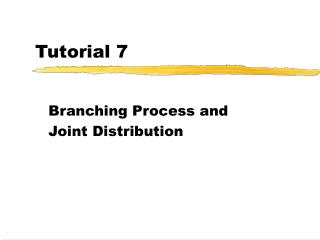# Tutorial 7 - PowerPoint PPT PresentationDownload PresentationTutorial 7

Tutorial 7Download Presentation## Tutorial 7

- - - - - - - - - - - - - - - - - - - - - - - - - - - E N D - - - - - - - - - - - - - - - - - - - - - - - - - - -
##### Presentation Transcript

1. Tutorial 7 Branching Process and Joint Distribution

2. Random sum of R.V.’s • Now Assume S = X1 + X2 +…+ XN where N is a R.V. ~ mN , Xi are i.i.d. ~ fX

3. Random sum of R.V.’s • But the z-transform of p.m.f. of N is • Put z = gX (t), then we have • If X is discrete,

4. Random sum of R.V.’s

5. Random sum of R.V.’s

6. Random sum of R.V.’s • This elegant result can be applied to the branching process.

7. Branching Process In a branching process: • each individual in a population can produce offspring of the same kind • During their lifetime, each individual can produce i new offspring with prob. Pi. • The no. of individuals initially present is called the size of the zero-th generation.

8. Branching Process • The population in the n-th generation is where Xj = no. of offspring produced by the j-th individual • Applying our previous result, we get

9. Branching Process - An Example • E.g. Given the size of zero-th generation=1 • Each individual can produce an offspring with prob. 0.6, i.e. {P0 , P1}= {0.4 , 0.6}. • We want to find the population distribution in the 3rd generation. • Let R.V. X = no. of offspring produced by an individual

10. Branching Process - An Example finally, we get {P0, P1} = {0.784, 0.216}. Note: if the zero-th generation size = 1, Try to prove it by yourself!

11. Joint Distribution • Let X, Y be two independent random variables that from 0 to 1. • Find fmin(x,y)(a) for 0 < a < 1 • P(min(X,Y)< a) = P(min(X,Y)< a , Y<X)+P(min(X,Y)< a , X<Y)

12. Joint Distribution • Let X1 and X2 be jointly continuous R.V. with joint prob. Density function f(x1, x2). • Suppose Y1=g1(X1, X2), Y2=g2(X1, X2)

13. Coordinate Transform • How to transform fX,Y(x,y) to fR,θ(r,θ) where

14. Coordinate Transform • J(x,y) = • And fX,Y(x,y) = fX(x) fY(y) = 1 • Thus, fR,θ(r,θ) = | J(x,y) |-1=r